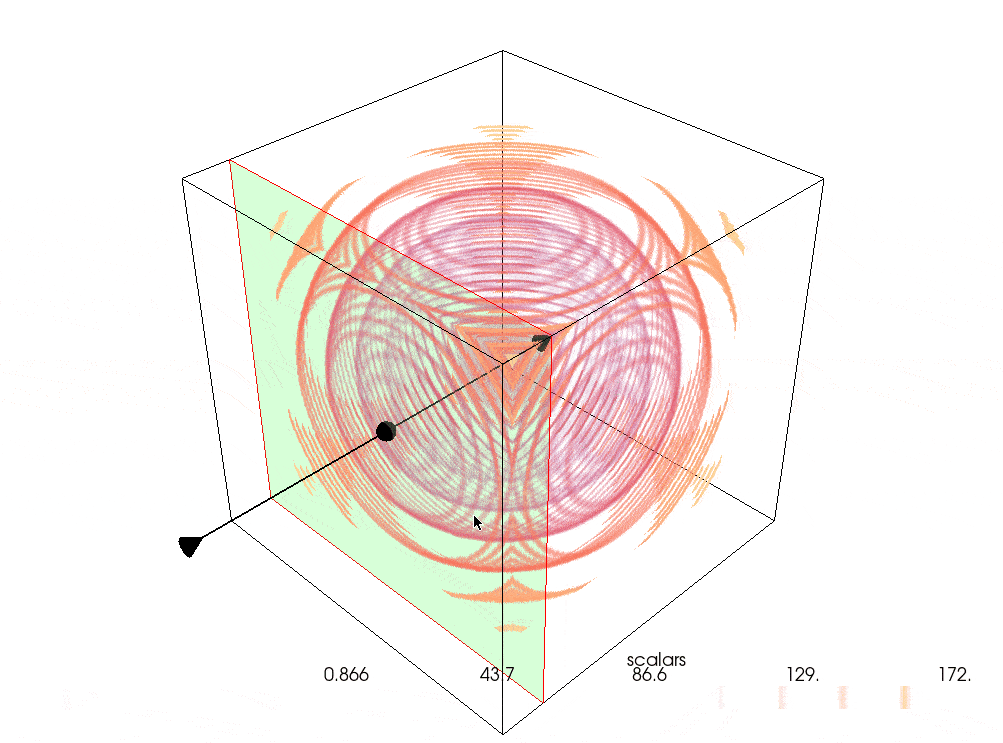# Clip Volume Widget#

If you have a structured dataset like a `pyvista.UniformGrid` or `pyvista.RectilinearGrid`, you can clip it using the `pyvista.Plotter.add_volume_clip_plane()` widget to better see the internal structure of the dataset.## Create the Dataset#

Create a dense `pyvista.UniformGrid` with dimensions ```(200, 200, 200)``` and set the active scalars to distance from the `center` of the grid.

```import numpy as np

import pyvista as pv

grid = pv.UniformGrid(dimensions=(200, 200, 200))
grid['scalars'] = np.linalg.norm(grid.center - grid.points, axis=1)
grid
```
UniformGridInformation
N Cells7880599
N Points8000000
X Bounds0.000e+00, 1.990e+02
Y Bounds0.000e+00, 1.990e+02
Z Bounds0.000e+00, 1.990e+02
Dimensions200, 200, 200
Spacing1.000e+00, 1.000e+00, 1.000e+00
N Arrays1
NameFieldTypeN CompMinMax
scalarsPointsfloat6418.660e-011.723e+02

## Generate the Opacity Array#

Create a banded opacity array such that our dataset shows “rings” at certain values. Have this increase such that higher values (values farther away from the center) are more opaque.

```opacity = np.zeros(100)
opacity[::10] = np.geomspace(0.01, 0.75, 10)
```

## Plot a Single Clip Plane Dataset#

Plot the volume with a single clip plane.

Reverse the opacity array such that portions closer to the center are more opaque.

```pl = pv.Plotter()
pl.show()
```## Plot Multiple Clip Planes#

Plot the dataset using the `pyvista.Plotter.add_volume_clip_plane()` with the output from `pyvista.Plotter.add_volume()` Enable constant interaction by setting the `interaction_event` to `'always'`.

Disable the arrows to make the plot a bit clearer and flip the opacity array.

```pl = pv.Plotter()
vol.prop.interpolation_type = 'linear'
vol,
normal='-x',
interaction_event='always',
normal_rotation=False,
)